Courses

# Partial Properties for Binary Solutions Civil Engineering (CE) Notes | EduRev

## Civil Engineering (CE) : Partial Properties for Binary Solutions Civil Engineering (CE) Notes | EduRev

The document Partial Properties for Binary Solutions Civil Engineering (CE) Notes | EduRev is a part of the Civil Engineering (CE) Course Thermodynamics.
All you need of Civil Engineering (CE) at this link: Civil Engineering (CE)

Partial Properties for Binary Solutions

The partial property is generally not amenable to ab initio computation from theory, but may be conveniently determined by suitably designed experiments that help obtain isothermal molar property of mixing (eqn. 6.17). Here we illustrate a set of results that derive for a binary mixture, and which may be applied to compute the relevant partial molar properties at any composition. Applying eqn. 6.10: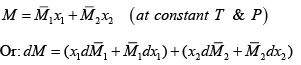......(6.18, 6.19)

Applying the Gibbs-Duhem equation (6.14) gives: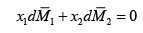......(6.20)

Now, since x+ x2= 1, dx1 = – dx2                    ......(6.21)

Using Eqns. 6.19 – 6.21: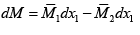......(6.22)

or :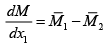......(6.23)

On solving eqns. 6.22 and 6.23 simultaneously: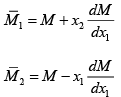......(6.24, 6.25)

If the molar property of the mixture M is available either from experiments or in analytical form, the partial molar properties may be estimated by applying the last two equations. Alternately, the experimental values of the mixture molar property (at a given T & P) may be plotted as a function of x1 as shown in fig.6.3.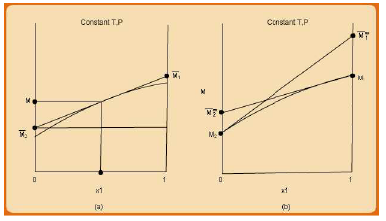Fig. 6.3. Graphical method of determination of partial molar properties for a binary solution

For determining the partial molar properties at a given concentration one may draw a tangent to the M vs. x1 curve, and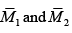obtain as the right and left intercepts on the y-axis. This may be evident on comparing with the equations 6.24 and 6.25.

Using eqn. 6.17, the molar property M of a mixture is also written as: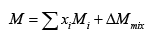......(6.26)

In general the most common form of analytical relation (obtained by fitting a polynomial to the experimentally determined values of isothermal ∆Mmix as a function of composition) is the well-known Redlich-Kister equation, which for a binary solution is given by: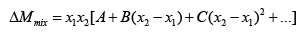......(6.27)

Where, A, B, C are temperature dependent and are determined from experimental measurements of ∆Mmix . For many practical applications the above equation is usually truncated to include only the terms corresponding to the parameters A and B. Fig. 6.4 shows the relation between the mixture enthalpy, the enthalpy change of mixing, the pure component and the partial molar enthalpies for a representative binary system.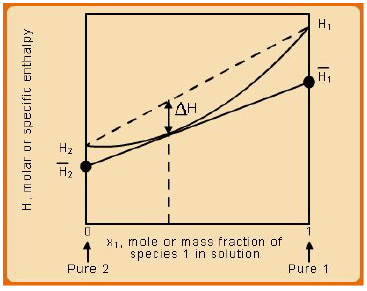Fig. 6.4. Schematic showing pure component and partial molar enthalpies for a binary solution.

Offer running on EduRev: Apply code STAYHOME200 to get INR 200 off on our premium plan EduRev Infinity!

## Thermodynamics

56 videos|92 docs|33 tests

,

,

,

,

,

,

,

,

,

,

,

,

,

,

,

,

,

,

,

,

,

;